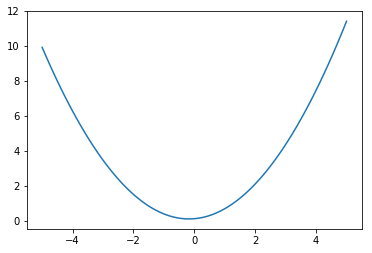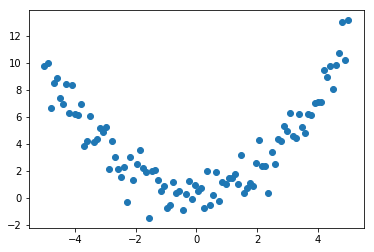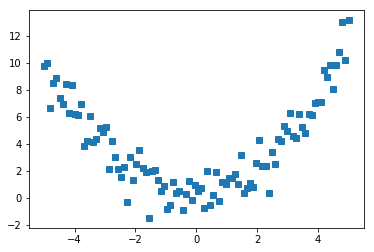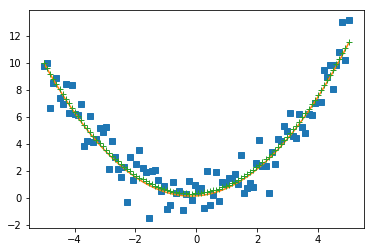# Example: Generating data and fitting a curve¶

In :
#  Our first step is to import any required modules.
import numpy as np
import matplotlib.pyplot as plt
%matplotlib inline
# embed the plots in the notebook


$1$. Generate a quadratic with random coefficients and plot.

In :
coef = np.random.random(3)
print(coef)
x = np.linspace(-5, 5, 100)
y0 = coef*x**2 + coef*x + coef
plt.plot(x, y0);

[ 0.42110478  0.14927527  0.14731543]$2$. Add in some Gaussian noise, representing random error in a measurement.

In :
sigma = 1.0
y = y0 + sigma*np.random.normal(size=len(x))
plt.scatter(x, y);$3$. Save the data to a text file. Here we will use NumPy instead of the core library.

In :
fname = "quadratic_with_noise.dat"
np.savetxt(fname, [x, y])


$4$. Read the data from a text file (just to show we can), and plot once more.

In :
x,y = np.loadtxt(fname)
plt.plot(x, y, 's');$5$. Now attempt to fit the data set using "curve_fit" from SciPy.

In :
from scipy.optimize import curve_fit
def func(x, a, b, c):
return a*x**2 + b*x + c
co, pcov = curve_fit(func, x, y)


$6$. Check that the fitted coefficients are reasonable estimates.

In :
print("%.4e\t%.4e\t%.4e" % tuple(co))   # The fitted coefficients
print("%.4e\t%.4e\t%.4e" % tuple(coef)) # The true coefficients

ytrue = coef*x**2 + coef*x + coef
yest = co*x**2 + co*x + co
plt.plot(x, y, 's')
plt.plot(x, ytrue, '-')
plt.plot(x, yest, '+');

4.1696e-01	1.5703e-01	3.3350e-01
4.2110e-01	1.4928e-01	1.4732e-01